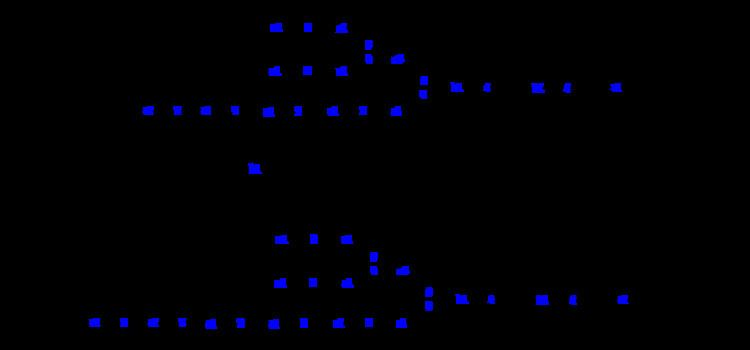ALG10 (enzyme class)

Updated on
 EC number  2.4.1.256BRENDA  BRENDA entryKEGG  KEGG entry IntEnz  IntEnz viewExPASy  NiceZyme viewMetaCyc  metabolic pathwayDolichyl-P-Glc:Glc2Man9GlcNAc2-PP-dolichol alpha-1,2-glucosyltransferase (EC 2.4.1.256, ALG10, Dol-P-Glc:Glc2Man9GlcNAc2-PP-Dol alpha-1,2-glucosyltransferase) is an enzyme with systematic name dolichyl beta-D-glucosyl phosphate:D-Glc-alpha-(1->3)-D-Glc-alpha-(1->3)-D-Man-alpha-(1->2)-D-Man-alpha-(1->2)-D-Man-alpha-(1->3)-(D-Man-alpha-(1->2)-D-Man-alpha-(1->3)-(D-Man-alpha-(1->2)-D-Man-alpha-(1->6))-D-Man-alpha-(1->6))-D-Man-beta-(1->4)-D-GlcNAc-beta-(1->4)-D-GlcNAc-diphosphodolichol 2-alpha-D-glucosyltransferase.

This enzyme catalyses the following chemical reaction:

which is:

dolichyl beta-D-glucosyl phosphate + D-Glc-alpha-(1->3)-D-Glc-alpha-(1->3)-D-Man-alpha-(1->2)-D-Man-alpha-(1->2)-D-Man-alpha-(1->3)-[D-Man-alpha-(1->2)-D-Man-alpha-(1->3)-[D-Man-alpha-(1->2)-D-Man-alpha-(1->6)]-D-Man-alpha-(1->6)]-D-Man-beta-(1->4)-D-GlcNAc-beta-(1->4)-D-GlcNAc-diphosphodolichol D-Glc-alpha-(1->2)-D-Glc-alpha-(1->3)-D-Glc-alpha-(1->3)-D-Man-alpha-(1->2)-D-Man-alpha-(1->2)-D-Man-alpha-(1->3)-[D-Man-alpha-(1->2)-D-Man-alpha-(1->3)-[D-Man-alpha-(1->2)-D-Man-alpha-(1->6)]-D-Man-alpha-(1->6)]-D-Man-beta-(1->4)-D-GlcNAc-beta-(1->4)-D-GlcNAc-diphosphodolichol + dolichyl phosphate

This eukaryotic enzyme performs the final step in the synthesis of the lipid-linked oligosaccharide.

Topics